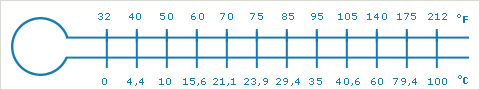## Temperature

Each syllable should be equally emphasised.• To convert the degree Celsius to the degree Fahrenheit, multiply by 9, divide by 5 and add 32.
• To covert the degree Fahrenheit to the degree Celsius, deduct 32, multiply by 5, and divide by 9.

Enter a number in either field, then click outside the text box.